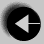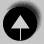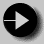## Type conversion

You can mix the types of values in your arithmetic expressions. char types will be treated as int. Otherwise where types of different size are involved, the result will usually be of the larger size, so a float and a double would produce a double result. Where integer and real types meet, the result will be a double.

There is usually no trouble in assigning a value to a variable of different type. The value will be preserved as expected except where;

• The variable is too small to hold the value. In this case it will be corrupted (this is bad).
• The variable is an integer type and is being assigned a real value. The value is rounded down. This is often done deliberately by the programmer.

Values passed as function arguments must be of the correct type. The function has no way of determining the type passed to it, so automatic conversion cannot take place. This can lead to corrupt results. The solution is to use a method called casting which temporarily disguises a value as a different type.

eg. The function sqrt finds the square root of a double.

```
int i = 256;
int root

root = sqrt( (double) i);```

The cast is made by putting the bracketed name of the required type just before the value. (double) in this example. The result of sqrt( (double) i); is also a double, but this is automatically converted to an int on assignment to root.Go to Arithmetic OperatorsGo to IndexGo to Comparison